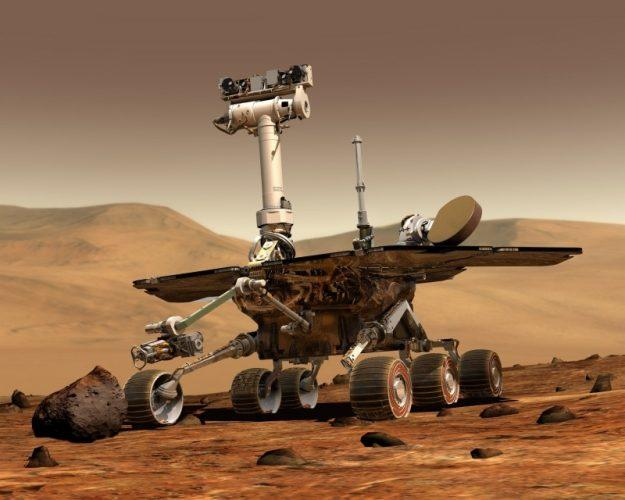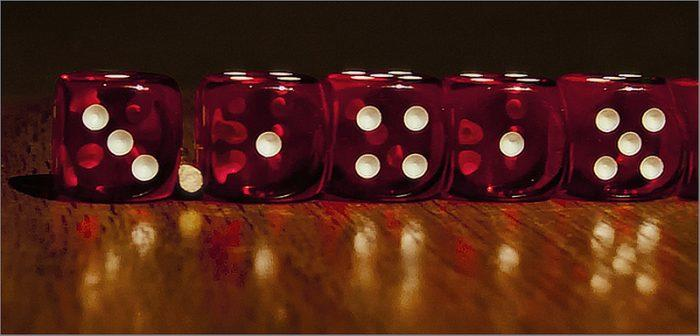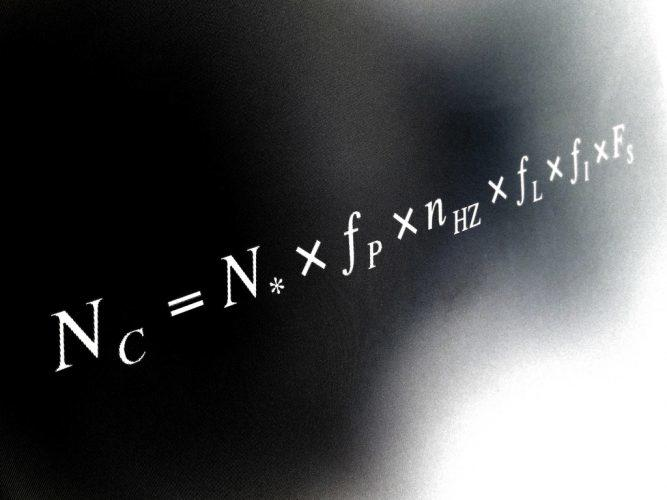Chapters

You might not be aware of it, but mathematics is all around us, all the time.

Much more than multiplication and division, fractions, algebra and geometry (which have still haunted much of our school curriculum), mathematics gives us a better understanding of the world we live in.

One day you'll thank your online math tutor.

When you take a closer interest in this science, you start to discover a number of mysteries and questions... Endless debates on the creation or the discovery of mathematics, impossible or unsolved equations, a fascination with certain numbers, unusual formulas, and so on.

Mathematical problems, theories and equations sometimes oscillating between religious and cultural myths and scientific facts.

You would think that maths is the subject of a precise and irrefutable logic allowing you, with the right equation of course, to solve any problem.

And yet ...The best Mathematics tutors available
1st lesson free!5 (15 reviews)
Syed
\$65
/h
1st lesson free!5 (5 reviews)
Davayne
\$13
/h
1st lesson free!Chintan
\$30
/h
1st lesson free!Zane
\$10
/h
1st lesson free!5 (6 reviews)
Laura
\$23
/h
1st lesson free!5 (4 reviews)
Stephen
\$25
/h
1st lesson free!Nicole
\$10
/h
1st lesson free!5 (12 reviews)
Namita
\$35
/h
1st lesson free!5 (15 reviews)
Syed
\$65
/h
1st lesson free!5 (5 reviews)
Davayne
\$13
/h
1st lesson free!Chintan
\$30
/h
1st lesson free!Zane
\$10
/h
1st lesson free!5 (6 reviews)
Laura
\$23
/h
1st lesson free!5 (4 reviews)
Stephen
\$25
/h
1st lesson free!Nicole
\$10
/h
1st lesson free!5 (12 reviews)
Namita
\$35
/h

## Mathematics: an invention or discovery of man?

Did mathematics derive from human thought or from a discovery?

Since ancient Greece, man has used mathematics, numbers and algebra to explore the physical world and understand the rules of nature.

Learning maths has also allowed numerous technological and scientific achievements such as:

• Decoding the orbits of the planets,
• Discovering the Higgs boson,
• Landing the Curiosity robot on Mars,
• And many others…Landing a robot on Mars? It's easy peasy with mathematics! Source: visualhunt

A documentary entitled "The Great Math Mystery" (2015) traces the philosophical debates about the universality and effectiveness of this science.

In the footsteps of the mathematical giants, Pythagoras, Galileo, Newton and Einstein, the feature highlights their fascinating discoveries and how these findings have evolved over time.

Pythagoras' theorems and mathematical equations, Newton's law of universal gravitation, the mysterious number Pi ... So many discoveries  made throughout history, which are still used today as points of reference. Scientific theories that are considered "the key to the Universe".

A captivating documentary that proves once again that mathematics is still an enigma that will not end up surprising and revealing!

## The 7 mathematical problems of the millennium

Did you know that there are still 7 mathematical problems that have never been solved!

They are called the 7 mathematical problems of the millennium.

In 1900 the mathematician D.Hilbert drews up a list of 23 unsolved mathematical problems to guide the mathematicians in their research.

100 years later, in 2000, the majority of maths experts found the solutions to these problems. Nevertheless, 7 still remain, described by some as the most difficult mathematical problems. Think you're a good problem solver?

To support research in science, especially in mathematics, a rich American, L. Clay, decided in 1999 to found the CMI (Clay Mathematics Institute) and to challenge mathematicians around the world.

If a mathematician manages to solve one of these problems, they could win £1 million! Yes, L.Clay is very rich!

Learn maths for £1 million? Tempting, isn't it?A million pounds to solve an equation? Does anyone have a calculator? | Source: visualhunt

Among the 7 mathematical problems never solved are:

• The Riemann hypothesis
• The Hodge conjecture
• The Birch and Swinnerton-Dyer conjecture
• The Navier-Stoke equations
• The Yang-Mills Equations
• P versus NP
• The Ramsey number
• Lychrel numbers and palindromes

After seeing all these problems, you will surely work harder on your maths homework and do better on your maths tests! Far beyond all that can be learned in school and even at University, extremely advanced knowledge is required before embarking on this adventure.

Very few mathematicians in the world have the opportunity to work on these equations and conjectures.

But as Albert Einstein says:

"We cannot solve our problems with the same thinking we used when we created them."

## The Numbers that Fascinate the World

Mathematics is a story of numbers. Among these, some are the subject of serious fascination. Mysterious numbers that we are trying to comprehend.

Looking for a math tutor, look no further. You will get all the maths help you need.

### Pi: the Number with Many Secrets

Pi is surely the most well-known mathematical constant. If you're struggling to remember your basic maths lessons, Pi is the value of the ratio between the circumference of a circle and its diameter.

More commonly summed up at the approximate value of 3.14, Pi has fascinated and intrigued mathematicians since antiquity for several reasons:

1. It is an irrational number: Pi can not be written as a fraction and its decimal places are infinite ...
2. A mysterious decimal sequence: currently, the most approximate decimal value of Pi has 1,241 billion digits. It was calculated by an extra-powerful computing software.
In fact, memorising the largest number of decimals of Pi is one of the records put in the Guinness Book of Records. It is currently held by a Chinese student who managed to recite 67,890 decimal places perfectly.
He must be a real pro in mental arithmetic!
3. An approximate but accurate figure: as it is impossible to define the exact value of Pi, its value remains approximate but, paradoxically, it allows us to make extremely accurate calculations.
4. Pi was used to build the pyramids: one of the greatest mysteries of this number could be that it has been proved that Pi would equal the ratio between the perimeter of the base of a pyramid and double the height. If we take, for example, the pyramid of Cheops, it has a ratio of 22/14 which corresponds to the approximation of Pi (which is 22/7) taking into account the double height.

Check here for aHow many numbers of Pi can you remember? | Source: visualhunt

### Phi: Gold at the Heart of the Universe

Phi, otherwise known as the golden number, is not to be confused with Pi.

The Golden ratio has a value that is more mythical. It could be, according to mathematicians, present in all things in our universe. Phi could be the factor of the proportions of everything around us: spaces, plants, and even human beings.

### The Whole Universe Would Obey the Law of Phi.

Closely linked to the famous Fibonacci sequence, the law of Phi would allow, for example, to draw shapes called "gold" with perfect proportions. Some even call the number the "divine proportion".

The most mysterious example is the work of Leonardo da Vinci and the famous "Vitruvian Man". De Vinci was the first to declare that the human body was composed of several parts that have the law of Phi as their common denominator.

If you measure the distance between the ground and the top of your head and divide it by the distance between the ground and your navel, you will get...wait for it...The value of Phi , about 1.618.

Unsettling, no?

This "divine" value can be found in many fields, such as:

• Art,
• Architecture,
• Music.

### The number 7: between belief and reality

Why is the number 7 also present in our past but also current cultures?

• The 7 Wonders of the World,
• The 7 colors of the rainbow,
• The 7 days of the week,

The boots of seven leagues, & c.

For several thousand years, the number 7 is has been thought to be lucky.

A figure that we come across a lot in our culture, and even religion, (there's a strong presence of the number 7 in the New and Old Testament).

And what about maths?

Mysteriously, the number 7 also has an important part to play in basic mathematics and geometry:

• 7 acute triangles divide into an obtuse triangle,
• The 7th is omnipresent in the triplets of Pythagoras,
• 7 types of "disaster" models in mathematics,
• 7 is a magic number,
• 7 is a prime number, and so on.

To this day no scientist has come up with a real explanation for the strong presence of the number 7 in mathematics. A mystery, a symbol, a myth ... Everyone is free to make their own interpretation.

Do you know the greatest mathematical mysteries?

## Drake's Mysterious Equation

Drake's equation, named after its creator, Franck Drake, is a serious mathematical theory to determine  the number of "possible" extraterrestrial civilisations in our galaxy.

One might think that this equation is science fiction, but not at all!

It's closely linked to such sciences as:

• Exobiology,
• Futuro-biology,
• Astro-sociology,
• And the well-known SETI (Search for Extra-Terrestrial Intelligence) project.

Published in 1961, Drake's formula still divides scientists and mathematicians, because the estimation of the factors which it is comprised of  is very uncertain ... We do not currently have all the knowledge necessary to grasp the equation.Drake's mathematical equation. | Source: visualhunt

It is the product of 7 factors (7 again...). The answer N represents the number of extraterrestrial civilisations that surround us.

For this reason, scientists must take into account the following factors (in order):

• The number of stars formed every year in our galaxy,
• The fraction of these stars which have planets,
• The average number of planets with potential for life per star,
• The fraction of these planets in which life appears,
• The fraction of these planets which has intelligent life,
• The fraction of these planets capable of communication,
• The average lifetime in years of these civilisations.

In 1961, Drake and his team managed to calculate a value for N which was 10. That means 10 extraterrestrial civilisations, able to communicate with us, exist in the Milky Way.

Mathematics, geometry, algorithms, probabilities, and trigonometry can become more than just a matter of academic interest.

Mathematics has had an incalculable number of secrets and mysteries since antiquity.

Will scientists succeed in cracking all the secret mathematical codes? Is mathematics a creation of man? Is the number 7 really lucky?

A subject that will never cease to amaze us!

Being interested in the mysteries of mathematics can stir up student's curiosity.

From an early age, children have access to many educational resources to learn maths while having fun (maths games, logic games, magic calculations, etc.), a sort of introduction to maths.

Through its mathematics school curriculum, the Board of Education proposes to accompany students from primary to higher education in various subjects of science and technology.

And why not make maths a profession, even a vocation? After A-levels, you have the opportunity to expand your knowledge of maths by pursuing a degree in engineering science or mathematics, for example.

But before that, you have to go through the most difficult part, work on your maths homework:

• Learn multiplication tables and theorems by heart ...
• Revise algebra, geometry, arithmetic, trigonometry
• Learn how to solve probabilities and equations
• Become an pro in Mental Arithmetic
• And of course do ALL of these maths lessons with a big smile!

To finish on an even funnier note, did you know that 2 scientists actually used maths to figure out the lead character in GOT? Tell that to your maths teacher!

Need a Mathematics tutor?

Enjoyed this article?5.00/5 - 1 vote(s)Loading...Jess